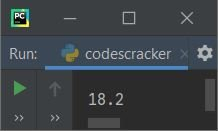# Operator precedence in Python with examples

In Python, operator precedence determines the order of operation in an expression with multiple operands and operators. Operator precedence provides the priority of operators that are used in expressions. That is, operator precedence determines which operation is carried out first, then which operation is carried out second, and so on.

That is, if there are multiple operands and operators in an expression, or if we need to create an expression with multiple operands and operators, then we need an operator precedence table to understand the precedence or priority of operators to do the thing in an accurate way. As a result, I created the operator precedence table to make things easier for you to understand.

## Operator Precedence Table

Take note that the operator in the top row has the most precedence, where the operator in the bottom (last) row has the lowest priority.

For example, operators in the 3rd row have higher precedence than operators in the 4th row but lower precedence than operators in the 2nd row. Similarly, operators in the 8th row have higher precedence than operators in the 9th row but lower precedence than operators in the 7th row.

Operator Name Syntax Precedence
** Exponent a ** b Highest Precedence
+, -, ˜ Unary Plus, Unary Minus, Bitwise NOT +a, -a, ˜a
*, /, //, % Multiplication, Division, Floor division, Modulus a*b, a/b, a//b, a%b
+, - Addition, Subtraction a + b, a - b
<<, >> Bitwise Left Shift, Bitwise Right Shift a << 2, a>>3
& Bitwise AND a & b
| Bitwise OR a | b
^ Bitwise XOR a ^ b
==, !=, >, >=, <, <= Comparison Operators a == b, a != b, a > b, a < b, a >= b, a <= b
is, is not Identity Operators a is b, a is not b
in, not in Membership Operators element in a, element not in a
not Logical NOT not(a > b)
and Logical AND a > b and a < c
or Logical OR a > b or a < c Lowest Precedence

Here is an example showing how the precedence of the operator determines the priority of the operation to be evaluated in an expression:

```a, b, c, d = 2, 3, 4, 5
res = a + b ** c / d
print(res)```

The output produced by the above program is shown in the snapshot given below:From the above example program, the expression gets evaluated in this way:

```  a + b ** c / d
= a + 3 ** 4 / d
= a + 81 / d
= a + 81 / 5
= a + 16.2
= 2 + 16.2
= 18.2```

I've not taken some of the operators in the expression given above, because if I do so, then the final answer will be in the form of "true" or "false." Therefore, to create a more understandable expression, I've done the things shown above. Now the expression given below uses most categories of operator. But before evaluating the expression, consider the statement given below:

`a, b, c, d, e, f = 2, 3, 4, 5, 6, 7`

initializes 2 to a, 3 to b, 4 to c, 5 to d, 6 to e, and 7 to f. That is, a = 2, b = 3, c = 4, d = 5, e = 6, and f = 7. Now let's evaluate the expression based on these variables:

```  a + b // c ** d != e is not f
= a + b // 4 ** 5 != e is not f
= a + b // 1024 != e is not f
= a + 3 // 1024 != e is not f
= a + 0 != e is not f
= 2 + 0 != e is not f
= 2 != e is not f
= 2 != 6 is not f
= True is not f
= True is not 7
= True```

Therefore, True is the final output, as you'll see after evaluating the above expression one by one while keeping operator precedence in mind.

Python Online Test

« Previous Tutorial Next Tutorial »